Test: Engineering Mathematics- 7

# Test: Engineering Mathematics- 7

Test Description

## 10 Questions MCQ Test GATE Computer Science Engineering(CSE) 2023 Mock Test Series | Test: Engineering Mathematics- 7

Test: Engineering Mathematics- 7 for Computer Science Engineering (CSE) 2023 is part of GATE Computer Science Engineering(CSE) 2023 Mock Test Series preparation. The Test: Engineering Mathematics- 7 questions and answers have been prepared according to the Computer Science Engineering (CSE) exam syllabus.The Test: Engineering Mathematics- 7 MCQs are made for Computer Science Engineering (CSE) 2023 Exam. Find important definitions, questions, notes, meanings, examples, exercises, MCQs and online tests for Test: Engineering Mathematics- 7 below.
Solutions of Test: Engineering Mathematics- 7 questions in English are available as part of our GATE Computer Science Engineering(CSE) 2023 Mock Test Series for Computer Science Engineering (CSE) & Test: Engineering Mathematics- 7 solutions in Hindi for GATE Computer Science Engineering(CSE) 2023 Mock Test Series course. Download more important topics, notes, lectures and mock test series for Computer Science Engineering (CSE) Exam by signing up for free. Attempt Test: Engineering Mathematics- 7 | 10 questions in 30 minutes | Mock test for Computer Science Engineering (CSE) preparation | Free important questions MCQ to study GATE Computer Science Engineering(CSE) 2023 Mock Test Series for Computer Science Engineering (CSE) Exam | Download free PDF with solutions
 1 Crore+ students have signed up on EduRev. Have you?
Test: Engineering Mathematics- 7 - Question 1

###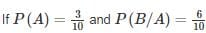where A and B are exhaustive events, then find P(B)?

Detailed Solution for Test: Engineering Mathematics- 7 - Question 1

Since A and B are exhaustive events

∴ P(A∪B) = 1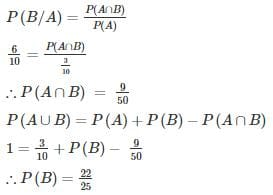Test: Engineering Mathematics- 7 - Question 2

### In an examination, 20% of the students have failed in Computer Networks, 30% of the students have failed in Computer Architecture and 10% in Computer Networks and Computer Architecture. If a student is selected at random, then what is the probability that the student has failed in at least one subject?

Detailed Solution for Test: Engineering Mathematics- 7 - Question 2

Let the number of students be 100.

P(N)→ probability that students failed in Computer Networks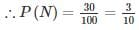P(A)→ probability that students failed in Computer Architecture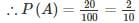P(N∩A)→ probability that the student has failed in both the subject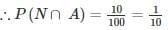P(NUA)→ probability that the  student has failed in atleast one subject

P(NUA) = P(N) + P(A) − P(N∩A)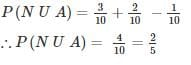Test: Engineering Mathematics- 7 - Question 3

### A coin is tossed 9 times. What is the probability that the outcome of only first two tosses is headed?

Detailed Solution for Test: Engineering Mathematics- 7 - Question 3

Required probability =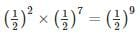Test: Engineering Mathematics- 7 - Question 4

A pair of dice is thrown. Find the probability of obtaining a sum of 8 or getting an even number on both the dice.

Detailed Solution for Test: Engineering Mathematics- 7 - Question 4

Let the events be defined as:

A: Obtaining a sum of 8

B: Getting an even number on both dice

P(A U B) = P(A) + P(B) – P (A ∩ B)

Now cases favourable to A are (3, 5) (5, 3) (2, 6) (6, 2) (4, 4)

So, P(A) = 5/36

Cases favourable to B: (2, 2), (2, 4), (2, 6), (4, 2), (4, 4), (4, 6), (6, 2), (6, 2), (6, 4), (6, 6).

P(B) = 9/36

Now, (2, 6) (6, 2) and (4, 4) are common to both events A and B

So, P (A ∩ B) = 3/36

⇒ P (A ∪ B) = (5/36) + (9/36) – (3/36) = 11/36

Test: Engineering Mathematics- 7 - Question 5

A bag contains 6 red, 5 blue balls, and another bag contains 5 red and 8 blue balls. A ball is drawn from the first bag without noticing the color is put in the second bag. A ball is drawn from the second bag. Find the probability that ball drawn is blue in color.

Detailed Solution for Test: Engineering Mathematics- 7 - Question 5

P(A)→ probability of getting a blue ball from the 2nd bag.

P(E1)→ probability of getting a red ball from the 1st bag.

P(E2)→ probability of getting a blue ball from the 1st bag.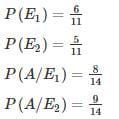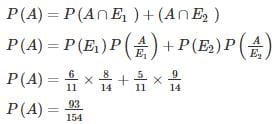*Answer can only contain numeric values
Test: Engineering Mathematics- 7 - Question 6

The chances of A, B, C becoming managers of a Testbook company are 4:2:3. The probability that the bonus scheme will be introduced if A, B, C become manager are 0.3, 0.5, 0.8 respectively.

If the bonus scheme has introduced, what is the probability that A is appointed as the manager of Testbook (answer up to 2 decimal place)?

Detailed Solution for Test: Engineering Mathematics- 7 - Question 6

P(X)→ probability that bonus scheme is introduced

P(A) → probability of A being a manager.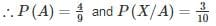P(B) → probability of B being a manager.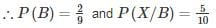P(C) → probability of C being a manager.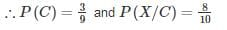P(X) = P (A ∩ X) + P (B ∩ X) + P (C ∩ X)

P(X) = P(A)P(X/A) + P(B)P(X/B) + P(C)P(X/C)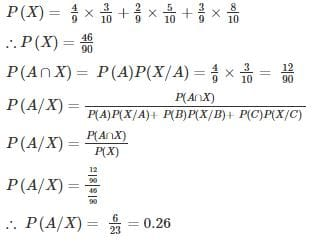*Answer can only contain numeric values
Test: Engineering Mathematics- 7 - Question 7

A speaks truth 3 out of 4 times. There is a chance that match can be won, drawn or lost but A reported that Shyam has won the match. Find the probability that his report was correct.

Detailed Solution for Test: Engineering Mathematics- 7 - Question 7

T: A speaks truth ⇒ P(T) = ¾

T̅ : A lies ⇒ P(T̅) = 1 - P(T) = ¼

There are three cases for matches. It can be won, drawn or lost.

The probability of winning a match, P (B/T) = 1/3

The probability of not winning a match, P(B,T̅) = 2/3

Using Baye’s theorem: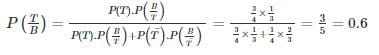*Answer can only contain numeric values
Test: Engineering Mathematics- 7 - Question 8

A and B throw a pair of dice alternately. A wins if he throws faces with sum 5 before B throws faces with the sum of 6 and B wins if he throws faces with the sum of 6 before A throw faces with the sum of 5. If A begins, the chances of winning for A is________.

Detailed Solution for Test: Engineering Mathematics- 7 - Question 8

The sum 5 can be obtained as follows:

(1, 4), (2, 3), (3, 2), (4, 1)

The probability of A is throwing 5 with two dice is

= 4/36 = 1/9

The probability of A is not throwing 5 is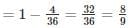The sum 6 can be obtained as follows:

(1, 5), (2, 4), (3, 3), (4, 2), (5, 1)

The probability of B is throwing 6 is

= 5/36

The probability of B is not throwing 6 is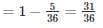Given that A begins,

Now A can win if he throws 5, in first chance,

(or) third Chace (b) fifth chance etc –

The chances of winning of A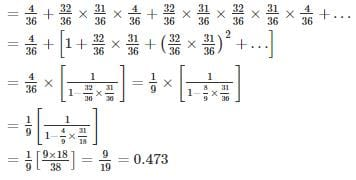*Answer can only contain numeric values
Test: Engineering Mathematics- 7 - Question 9

An anti-aircraft gun can take a maximum of 4 shots at an enemy plane moving away from it. The probabilities of hitting the plane at the first, second, third and fourth shot are 0.4, 0.3, 0.2 and 0.1 respectively. The probability that the gun hits the plane is_____

Detailed Solution for Test: Engineering Mathematics- 7 - Question 9

Let p1 = 0.4, p2 = 0.3, p3 = 0.2 and p4 = 0.1

P(the gun hits the plane) = P(the plane is hit at least once)

= 1 – P(the plane is hit in none of the shots)

= 1− (1−p1) (1−p2) (1−p3) (1−p4)
= 1− (0.6 × 0.7 × 0.8 × 0.9)
= (1− 0.3024) = 0.6976

Test: Engineering Mathematics- 7 - Question 10

What is the probability of getting more than one tail given that at least one coin is head when 4 coins are tossed?

Detailed Solution for Test: Engineering Mathematics- 7 - Question 10

P(A)→ probability of getting more than one tail

∴ P(A) = 11/16

P(B)→ probability of getting at least one tail

∴ P(B) = 15/16

P(A ∩ B)→ probability of getting more than one tail and at least one is head.

∴ P(A ∩ B) = 10/16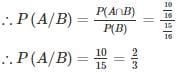## GATE Computer Science Engineering(CSE) 2023 Mock Test Series

150 docs|215 tests
Information about Test: Engineering Mathematics- 7 Page
In this test you can find the Exam questions for Test: Engineering Mathematics- 7 solved & explained in the simplest way possible. Besides giving Questions and answers for Test: Engineering Mathematics- 7, EduRev gives you an ample number of Online tests for practice

## GATE Computer Science Engineering(CSE) 2023 Mock Test Series

150 docs|215 tests(Scan QR code)